Next: Relativistic particle dynamics Up: Relativity and electromagnetism Previous: Potential due to a

## Fields due to a moving charge

Although the fields generated by a uniformly moving charge can be calculated from the expressions (1525) and (1526) for the potentials, it is simpler to calculate them from first principles.

Let a charge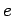, whose position vector at time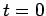is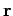, move with uniform velocity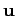in a framewhose-axis has been chosen in the direction of. We require to find the field strengths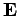and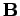at the event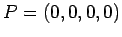. Let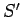be that frame in standard configuration within which the charge is permanently at rest. In, the field is given by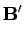(1528)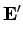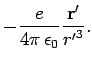(1529)

This field must now be transformed into the frame. The direct method, using Eqs. (1512)-(1515), is somewhat simpler here, but we shall use a somewhat indirect method because of its intrinsic interest.

In order to express Eq. (1528) and (1529) in tensor form, we need the electromagnetic field tensoron the left-hand side, and the position 4-vector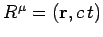and the scalar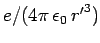on the right-hand side. (We regard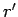as an invariant for all observers.) To get a vanishing magnetic field in, we multiply on the right by the 4-velocity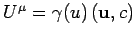, thus tentatively arriving at the equation(1530)

Recall thatand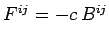. However, this equation cannot be correct, because the antisymmetric tensorcan only be equated to another antisymmetric tensor. Consequently, let us try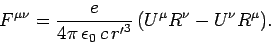(1531)

This is found to give the correct field atin, as long as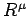refers to any event whatsoever at the charge. It only remains to interpret (1531) in. It is convenient to choose forthat event at the charge at which(not the retarded event). Thus,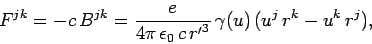(1532)

giving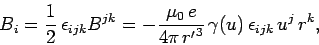(1533)

or(1534)

Likewise,(1535)

or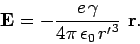(1536)

Lastly, we must find an expression for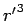in terms of quantities measured inat time. If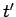is the corresponding time inat the charge, we have(1537)

Thus,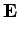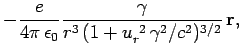(1538)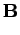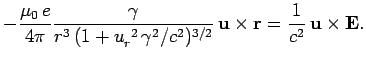(1539)

Note thatacts in line with the point which the charge occupies at the instant of measurement, despite the fact that, owing to the finite speed of propagation of all physical effects, the behaviour of the charge during a finite period before that instant can no longer affect the measurement. Note also that, unlike Eqs. (1525) and (1526), the above expressions for the fields are not valid for an arbitrarily moving charge, not can they be made valid by merely using retarded values. For whereas acceleration does not affect the potentials, it does affect the fields, which involve the derivatives of the potential.

For low velocities,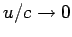, Eqs. (1538) and (1539) reduce to the well-known Coulomb and Biot-Savart fields. However, at high velocities,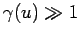, the fields exhibit some interesting behaviour. The peak electric field, which occurs at the point of closest approach of the charge to the observation point, becomes equal to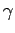times its non-relativistic value. However, the duration of appreciable field strength at the pointis decreased. A measure of the time interval over which the field is appreciable is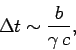(1540)

whereis the distance of closest approach (assuming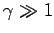). Asincreases, the peak field increases in proportion, but its duration goes in the inverse proportion. The time integral of the field is independent of. As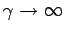, the observer atsees electric and magnetic fields which are indistinguishable from the fields of a pulse of plane polarized radiation propagating in the-direction. The direction of polarization is along the radius vector pointing towards the particle's actual position at the time of observation.Next: Relativistic particle dynamics Up: Relativity and electromagnetism Previous: Potential due to a
Richard Fitzpatrick 2006-02-02